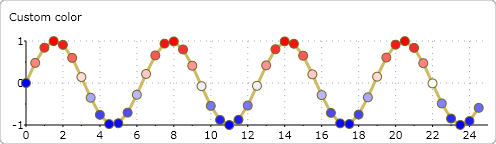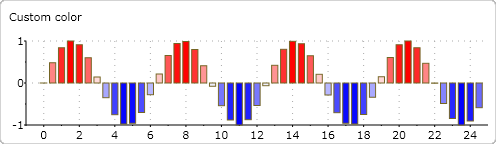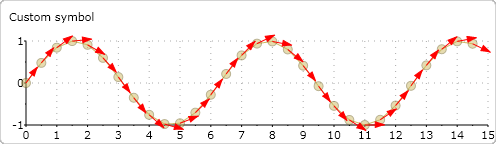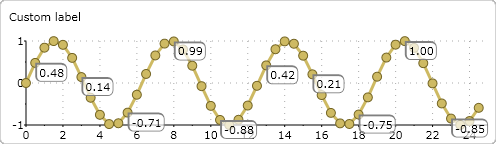﻿ Data Point Customization for ComponentOne Chart# Data Point Customization for ComponentOne Chart

The C1Chart control for Silverlight/WPF allows for extensive customization. One of the most flexible techniques uses the DataSeries.PlotElementLoaded event to override the default appearance and behavior of chart elements created by the chart. The PlotElementLoaded event fires after the plot element has been loaded on the chart canvas. By overriding chart elements we can customize the visual representation of the data points beyond standard settings to get the exact result desired. We can even add our own custom elements to the chart surface such as arrows and labels.

The PlotElement is responsible for the visual representation of the data points on the chart. Its specific type depends on the chart type. For column charts, elements are columns; for pie charts elements are pie slices and so on. Each PlotElement has a DataPoint property which provides information about the data point represented by the element.

The following sections illustrate different ways to customize the plot elements.

## Default Appearance

Let's start with a simple line chart with symbols at each point. The snippet of code below illustrates how easy it is to create basic charts:

``````  void DefaultAppearance()
{
}
XYDataSeries CreateDataSeries(int npts)
{
double[] x = new double[npts];
double[] y = new double[npts];
for (int i = 0; i < npts; i  )
{
x[i] = 0.5*i; y[i] = Math.Sin(x[i]);
}
return new XYDataSeries() { XValuesSource = x, ValuesSource = y };
}

``````

The resulting chart looks like this:

## Customizing Colors

Next, let's customize the chart by filling the points with colors that depend on the value being plotted. To achieve this, we will assign custom brushes to the PlotElement.Fill property in the PlotElementLoaded event handler:

``````void CustomDataPointColor()
{
var ds = CreateDataSeries(50);
{
var pe = (PlotElement)sender;
var dp = pe.DataPoint;
if (!(pe is Lines)) // skip connecting lines
{
// fill the symbol with a color calculated from the data value
pe.Fill = GetBrush(Colors.Blue, Colors.White, Colors.Red, dp.Value);
}
}
}

``````

The resulting chart looks like this:The GetBrush method used above creates a brush by mixing colors in the proportion defined by the parameter. Of course, you can use any other method to color the points. For example, you could highlight points within certain ranges or generate colors based on parameters that are not being charted (thereby adding a new dimension to the chart).

Notice that the code listed above is not specific to symbol charts. If you change the chart type to column for example, you would get different elements with the same custom colors:## Custom Size

Another common customization is using symbols of different sizes. This can be accomplished by applying a scale transformation to each plot element. The following code modifies the size of the points depending on the value being plotted. Notice that the transform origin is also changed to keep the center of the symbol on the same place.

``````void CustomDataPointSize()
{
var ds = CreateDataSeries(50);
{
var pe = (PlotElement)s;
var dp = pe.DataPoint;
if (!(pe is Lines)) // skip connecting lines
{
// apply simple scale transformation depending on data point value
double scale = 1   0.4 * dp.Value;
pe.RenderTransform = new ScaleTransform() { ScaleX = scale, ScaleY = scale };
pe.RenderTransformOrigin = new Point(0.5, 0.5);
}
};
}

``````

The resulting chart looks like this:

## Custom Symbols

Changing colors and sizes was quite simple. All we had to do was use modify existing WPF/Silverlight objects used to represent the data points.

But you are not restricted to modifying the elements created by the chart. You can also create new custom elements to represent the data points, replacing the default ones entirely.

The code below illustrates this technique using arrows to represent each data point. The arrows point in the direction of the tangent at the point.

``````  void CustomDataPointSymbol()
{
var ds = CreateDataSeries(30);
ds.ConnectionStrokeThickness = 1;

{
var pe = (PlotElement)sender;
var dp = pe.DataPoint as XYDataSeries.XYDataPoint;
if (!(pe is Lines)) // skip connecting lines
{
pe.Opacity = 0.5;
var pnl = pe.Parent as Panel;
if (pnl != null)
{
var x = (double)dp.X;

// find angle of tangent
var angle = Math.Atan( Math.Cos(x)/ GetAspectRatio(chart));

// create arrow
var arrow = CreateArrow( angle, 20);
arrow.Fill = arrow.Stroke = new SolidColorBrush(Colors.Red);

// set position (base) of arrow
Canvas.SetLeft(arrow, pe.Center.X);
Canvas.SetTop(arrow, pe.Center.Y);

// add the arrow to the plot
}
}
};
}

``````

The resulting chart looks like this:The code above has some points of interest:

• The new element is added to the chart using the original element's Parent property, and is positioned on the chart using the standard Canvas.SetLeft and Canvas.SetTop methods.
• This sample adds a new element for each data point (arrow) and leaves the original element (circle) on the chart. Removing the original element would be easy, you would simply call `pnl.Children.Remove(pe)`.
• The equation of the original function is known so it is easy to obtain the angle of the tangent at each point. As in the previous example, the angle could also be used to represent values not currently shown, adding a new dimension to the chart.
• The equation for the angle uses data coordinates, but the arrow is rendered using screen coordinates. The GetAspectRatio method finds the coefficient required to move from data to screen coordinates.
• The CreateArrow method builds arrows in code as geometric elements.
• The CreateArrow and GetAspectRatio methods are not included in the code snippet above but you can find them in the sample project attached to this article.

Now we know how to add custom symbols to the chart. Another common type of plot element are data annotations (or labels). You can easily create chart labels in XAML using the DataSeries.PointLabelTemplate, but creating data labels in code is much more flexible. Here is some code that creates one label for each group of five data points:

``````  void CustomDataPointLabel()
{
var ds = CreateDataSeries(50);

{
var pe = (PlotElement)sender;
var dp = pe.DataPoint;
if (!(pe is Lines)  // skip connecting lines
&& (dp.PointIndex % 5) == 1 ) // each 5-th point
{
var pnl = pe.Parent as Panel;
if (pnl != null)
{
var tb = new TextBlock() { Text = dp.Value.ToString("0.00") };
var bdr = new Border()
{
Background = new SolidColorBrush(Colors.White) { Opacity=0.7},
BorderBrush = new SolidColorBrush(Colors.Gray),
BorderThickness = new Thickness(2, 2, 1, 1),
};
// set label position
Canvas.SetLeft(bdr, pe.Center.X);
Canvas.SetTop(bdr, pe.Center.Y);
}
}
};
}

``````

The resulting chart looks like this:The DataSeries.PlotElementLoaded event can be used to customize or create new data points. It can also be used to create animations or to attach behaviors to the plot elements.